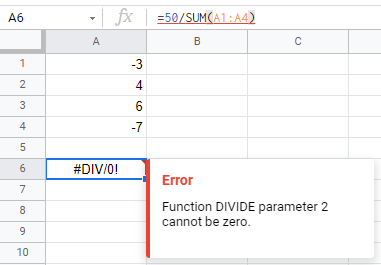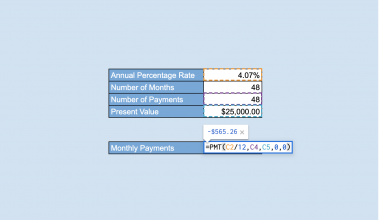# How to Fix ‘#DIV/0!’ Error in Google Sheets

The #DIV/0! error in Google Sheets appears when the formula divides a number with zero or any denominator that has a value of zero. As this does not make sense mathematically, hence Google Sheets would return an error.

It is no surprise that formula parse errors may arise from time to time when you create formulas in Google Sheets. One of the most common formula parse errors would be the #DIV/0! error.

This is a simple error to fix and find out what caused it. Let’s move on to find out how! 🦾

## How to Fix the #DIV/0! Error in Google Sheets

There are some recommended ways to fix the #DIV/0! error in Google Sheets. We will be looking at some examples to understand what causes the #DIV/0! error would appear and how to fix it.

#### Example 1:

The most common source of the #DIV/0! error is a blank cell that has gone undiscovered.As you can see, cell D4’s formula involved dividing B4 with C4. However, C4 is an empty cell, causing the value to be zero.

To fix this, you need to populate the cell selected for the formula.There you go, problem fixed!

#### Example 2:

Another common cause for #DIV/0! error to appear is when the formula consists of invalid data.The error is being thrown because the data used in the `AVERAGE` function is invalid. The formula requires a numeric value to work; however, A2:A5 contains texts.

Thus, make sure the data or range selected for the function are numeric values.

#### Example 3:

Even if the denominator inputted in the formula is not blank, if the value is zero, the formula will still return the #DIV/0! error.As you can see, the SUM of A1:A4 is equivalent to zero. This caused the error to appear.

A quick fix would be to highlight the formula used within the formula to ensure that the denominator is not zero.Google Sheets would assist you with a preview of the formula within another formula.

#### Example 4:

Another reason for the #DIV/0! error to appear would be when the formula range selected contains the same error as well.Since D4 has an error, and it is part of the selected formula range, it would cause the final formula to return an error.

Once we have fixed the original error, the final formula would work properly.

## Using  IFERROR Function in Google Sheets

You must be thinking, is there a way to beautify this error if the #DIV/0! Would be present either way.

The answer is yes! We can hide the error by surrounding it with another function with is the `IFERROR` function in Google Sheets.

Instead of showing an error message, it assigns a value to show whenever an error is found. Check out our in-depth tutorial on the `IFERROR` function if you are interested!So instead of showing the #DIV/0! error, we can customize a message instead.Let’s learn how to do this step-by-step.

1. First, simply select the cell you would like to input your formula in. In this example, it would be D4.1. Then, we would start the formula with an equal sign `=`, followed by the function name, `IFERROR`.1. The cost per price for SF3254 shall be computed by dividing B4 with C4. We would then add a comma `,` to separate the attributes.1. Lastly, we will customize what value will return if there is a #DIV/0! error. In this example, we choose to leave a “No Stock” message to imply that no more stocks are left.1. Your outcome would look like this:You may make a copy of the spreadsheet using the link attached below and try it for yourself:

There you go! Now you can easily identify the origin of the #DIV/0! error in Google Sheets and how to fix them. If you are interested in how to fix other formula parse errors as well, check out our tutorial on the five most common formula parse errors!Our goal this year is to create lots of rich, bite-sized tutorials for Google Sheets users like you. If you liked this one, you'll love what we are working on! Readers receive ✨ early access ✨ to new content.

##### You May Also Like## How to Sort Data in Google Sheets

In this article, we’ll learn how to sort data in Google Sheets. We’ll see the built-in functionality in…## How to Use IFERROR Function in Google Sheets

The IFERROR function in Google Sheets is used to effectively manage errors. Instead of showing an error message,…## How to Use PROPER Function in Google Sheets

The PROPER function in Google Sheets is useful if you want to capitalize words on a given text…## How to Use the PMT Function in Google Sheets

The PMT function in Google Sheets is used to calculate the periodic payment for an annuity investment based…